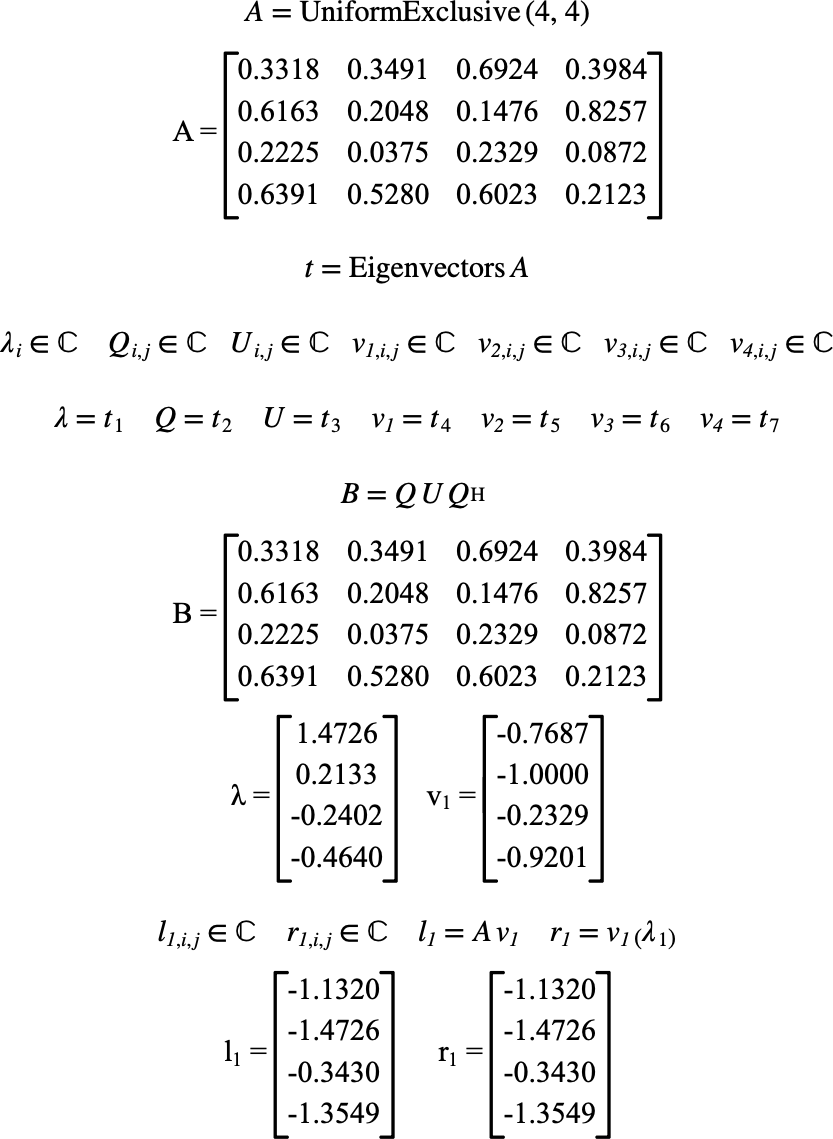# $$\text{Eigenvectors}$$¶

You can use the $$\text{Eigenvectors}$$ function to calculate eigenvectors, eigenvalues and Schur decomposition of the matrix.

You can use the \eigenvectors backslash command to insert this function.

The following variants of this function are available:

• $$\text{tuple } \text{eigenvalues} \left ( \text{<matrix>} \right )$$

The $$\text{Eigenvectors}$$ function returns a tuple that will contain:

• A column matrix holding the eigenvalues of the matrix.

• The Q unitary matrix matrix from the Schur decomposition.

• The U upper triangular matrix that represents the Schur decomposition.

• Zero or more column matrices representing the eigenvectors corresponding to the supplied eigenvalues.

Figure 125 shows the basic use of the $$\text{Eigenvectors}$$ function.Figure 125 Example Use Of The Eigenvectors Function